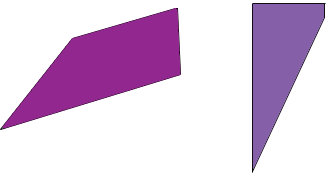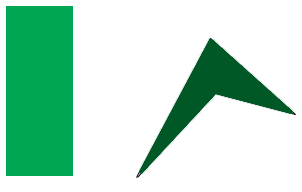SEARCH HOMEMath Central Quandaries & QueriesQuestion from Eva: Are all quadrilaterals the same?Hi Eva,

It depends on what you mean by "the same".

A quadrilateral is a closed plane figure bounded by four line segments. Here are two examples.and here are another twoAre they the same? They are all closed plane figure bounded by four line segments but they are quite different shapes. The side lengths are different and the interior angles are different. I expect you would call them different quadrilaterals.

PennyMath Central is supported by the University of Regina and The Pacific Institute for the Mathematical Sciences.